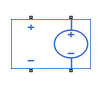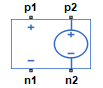# PVCVS

Polynomial voltage-controlled voltage source

•Libraries:
Simscape / Electrical / Additional Components / SPICE Sources

## Description

The PVCVS (Polynomial Voltage-Controlled Voltage Source) block represents a voltage source whose output voltage value is a polynomial function of the voltage across the input ports. The following equations describe the voltage across the source as a function of time:

• If you specify an n-element vector of polynomial coefficients for the Polynomial coefficients parameter:

`${V}_{out}=p\left(0\right)+p\left(1\right)*{V}_{in}+...+p\left(n-1\right)*{V}_{in}^{n-1}+p\left(n\right)*{V}_{in}^{n}$`

• If you specify a scalar coefficient for the Polynomial coefficients parameter:

`${V}_{out}=p*{V}_{in}$`

where:

• Vin is the voltage across the input ports.

• p is the Polynomial coefficients parameter value.

## Ports

Refer to the figure for port locations.### Conserving

expand all

Electrical conserving port associated with the PVCVS positive voltage of the controlling input.

Electrical conserving port associated with the PVCVS negative voltage of the controlling input.

Electrical conserving port associated with the PVCVS positive output voltage.

Electrical conserving port associated with the PVCVS negative output voltage.

## Parameters

expand all

The polynomial coefficients that relate the input voltage to the output voltage, as described in the preceding section.

## Version History

Introduced in R2008a